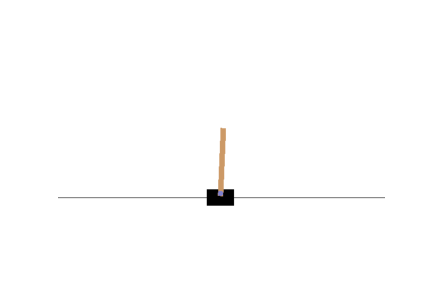# Training with Policy Gradients

• Now let us proceed to train the neural network using policy gradients concept.

• For transparency, let us clear the session and create the model here and train it using policy gradients.

• Then we shall see the improvement that the policy gradients caused.

INSTRUCTIONS
• Let us first clear the session:

``````keras.backend.clear_session()
``````
• Set the `tf` and `np` seeds:

``````tf.random.set_seed(42)
np.random.seed(42)
``````
• Set the number of environments `n_episodes_per_update` to `10`.

``````n_episodes_per_update = 10
``````
• Set the number of iterations to `150`.

``````n_iterations = 150
``````
• Set the number of steps per episode to `200`:

``````n_max_steps = 200
``````
• Set the discount rate to `0.95`.

``````discount_rate = 0.95
``````
• Let us set the input shape to be 4, since we shall feed all the values of `obs`:

``````n_inputs = 4
``````
• Initialize the `optimizer` to `keras.optimizers.Adam(lr=0.01)`:

``````optimizer = keras.optimizers.Adam(lr=0.01)
``````
• Initialize the `loss_fn` function to be `keras.losses.binary_crossentropy`:

``````loss_fn = keras.losses.binary_crossentropy
``````
• Define the `nn_policy_gradients()` function. In this function, we apply the policy gradients algorithm while training the neural networks:

``````def nn_policy_gradient(model, n_iterations, n_episodes_per_update, n_max_steps, loss_fn):
env = gym.make("CartPole-v1")
env.seed(42);

for iteration in range(n_iterations):
all_rewards, all_grads = play_multiple_episodes(
env, n_episodes_per_update, n_max_steps, model, loss_fn)
total_rewards = sum(map(sum, all_rewards))                     # Not shown in the book
print("\rIteration: {}, mean rewards: {:.1f}".format(          # Not shown
iteration, total_rewards / n_episodes_per_update), end="") # Not shown
all_final_rewards = discount_and_normalize_rewards(all_rewards,
discount_rate)
all_mean_grads = []
for var_index in range(len(model.trainable_variables)):
mean_grads = tf.reduce_mean(
[final_reward * all_grads[episode_index][step][var_index]
for episode_index, final_rewards in enumerate(all_final_rewards)
for step, final_reward in enumerate(final_rewards)], axis=0)
all_mean_grads.append(mean_grads)
optimizer.apply_gradients(zip(all_mean_grads, model.trainable_variables))

return model

env.close()
``````

Here, for iteration,

• call the `play_multiple_episodes` function which makes the agent play multiple number of episodes(here 10 episodes, each episode having 200 maximum steps). For each step, we store the rewards and gradients. Then for each episode, all the rewards and gradients(for all the steps in that episode) are stored in `all_rewards` and `all_gradients`, and returned.

• then, we compute the total rewards and calculate the mean rewards. Next, we discount and normalize the rewards.

• then we calculate the loss between the weighted gradients and the final rewards, to calculate the mean gradients. Then we apply these mean gradients. Finally, close the environment at the end of all iterations.

• Now, let us build the neural network as follows:

``````model = keras.models.Sequential([
keras.layers.Dense(5, activation="elu", input_shape=[n_inputs]),
keras.layers.Dense(1, activation="sigmoid"),
])
``````
• Call the function:

``````model = nn_policy_gradient(model, n_iterations, n_episodes_per_update, n_max_steps, loss_fn)
``````

This might take around 5-10 mins.

Let us see the visual of the cartpole now, after training the neural network using policy gradients:Now this looks as if the neural network managed to learn a better policy by itself. The pole is not much wobbly and thus we would expect to be improvement in the minimum, maximum and mean of the steps the cartpole game played by agent.

• Let us again call the `basic_policy_untrained` function, which performs the inference using the model we now trained based on policy gradients.

``````totals = []
for episode in range(20):
print("Episode:",episode)
episode_rewards = 0
obs = env.reset()
for step in range(200):
action = basic_policy_untrained(obs)
obs, reward, done, info = env.step(action)
episode_rewards += reward
if done:
break
totals.append(episode_rewards)

np.mean(totals), np.std(totals), np.min(totals), np.max(totals)
``````

Wow! We see the maximum steps are 200, meaning that the agent has won the game at least once. Also, there is a significant improvement in the minimum and the average number of steps the agent managed to balance the pole. That's a great improvement achieved using policy gradients!

No hints are availble for this assesment

Answer is not availble for this assesment

Note - Having trouble with the assessment engine? Follow the steps listed here